### (function(){for(var g="function"==typeof Object.defineProperties?Object.defineProperty:function(b,c,a){if(a.get||a.set)throw new TypeError("ES3 does not support getters and setters.");b!=Array.prototype&&b!=Object.prototype&&(b[c]=a.value)},h="undefined"!=typeof window&&window===this?this:"undefined"!=typeof global&&null!=global?global:this,k=["String","prototype","repeat"],l=0;l<k.length-1;l++){var m=k[l];m in h||(h[m]={});h=h[m]}var n=k[k.length-1],p=h[n],q=p?p:function(b){var c;if(null==this)throw new TypeError("The 'this' value for String.prototype.repeat must not be null or undefined");c=this+"";if(0>b||1342177279<b)throw new RangeError("Invalid count value");b|=0;for(var a="";b;)if(b&1&&(a+=c),b>>>=1)c+=c;return a};q!=p&&null!=q&&g(h,n,{configurable:!0,writable:!0,value:q});var t=this;function u(b,c){var a=b.split("."),d=t;ain d||!d.execScript||d.execScript("var "+a);for(var e;a.length&&(e=a.shift());)a.length||void 0===c?d[e]?d=d[e]:d=d[e]={}:d[e]=c};function v(b){var c=b.length;if(0<c){for(var a=Array(c),d=0;d<c;d++)a[d]=b[d];return a}return[]};function w(b){var c=window;if(c.addEventListener)c.addEventListener("load",b,!1);else if(c.attachEvent)c.attachEvent("onload",b);else{var a=c.onload;c.onload=function(){b.call(this);a&&a.call(this)}}};var x;function y(b,c,a,d,e){this.h=b;this.j=c;this.l=a;this.f=e;this.g={height:window.innerHeight||document.documentElement.clientHeight||document.body.clientHeight,width:window.innerWidth||document.documentElement.clientWidth||document.body.clientWidth};this.i=d;this.b={};this.a=[];this.c={}}function z(b,c){var a,d,e=c.getAttribute("data-pagespeed-url-hash");if(a=e&&!(e in b.c))if(0>=c.offsetWidth&&0>=c.offsetHeight)a=!1;else{d=c.getBoundingClientRect();var f=document.body;a=d.top+("pageYOffset"in window?window.pageYOffset:(document.documentElement||f.parentNode||f).scrollTop);d=d.left+("pageXOffset"in window?window.pageXOffset:(document.documentElement||f.parentNode||f).scrollLeft);f=a.toString()+","+d;b.b.hasOwnProperty(f)?a=!1:(b.b[f]=!0,a=a<=b.g.height&&d<=b.g.width)}a&&(b.a.push(e),b.c[e]=!0)}y.prototype.checkImageForCriticality=function(b){b.getBoundingClientRect&&z(this,b)};u("pagespeed.CriticalImages.checkImageForCriticality",function(b){x.checkImageForCriticality(b)});u("pagespeed.CriticalImages.checkCriticalImages",function(){A(x)});function A(b){b.b={};for(var c=["IMG","INPUT"],a=[],d=0;d<c.length;++d)a=a.concat(v(document.getElementsByTagName(c[d])));if(a.length&&a.getBoundingClientRect){for(d=0;c=a[d];++d)z(b,c);a="oh="+b.l;b.f&&(a+="&n="+b.f);if(c=!!b.a.length)for(a+="&ci="+encodeURIComponent(b.a),d=1;d<b.a.length;++d){var e=","+encodeURIComponent(b.a[d]);131072>=a.length+e.length&&(a+=e)}b.i&&(e="&rd="+encodeURIComponent(JSON.stringify(B())),131072>=a.length+e.length&&(a+=e),c=!0);C=a;if(c){d=b.h;b=b.j;var f;if(window.XMLHttpRequest)f=new XMLHttpRequest;else if(window.ActiveXObject)try{f=new ActiveXObject("Msxml2.XMLHTTP")}catch(r){try{f=new ActiveXObject("Microsoft.XMLHTTP")}catch(D){}}f&&(f.open("POST",d+(-1==d.indexOf("?")?"?":"&")+"url="+encodeURIComponent(b)),f.setRequestHeader("Content-Type","application/x-www-form-urlencoded"),f.send(a))}}}function B(){var b={},c;c=document.getElementsByTagName("IMG");if(!c.length)return{};var a=c;if(!("naturalWidth"in a&&"naturalHeight"in a))return{};for(var d=0;a=c[d];++d){var e=a.getAttribute("data-pagespeed-url-hash");e&&(!(e in b)&&0<a.width&&0<a.height&&0<a.naturalWidth&&0<a.naturalHeight||e in b&&a.width>=b[e].o&&a.height>=b[e].m)&&(b[e]={rw:a.width,rh:a.height,ow:a.naturalWidth,oh:a.naturalHeight})}return b}var C="";u("pagespeed.CriticalImages.getBeaconData",function(){return C});u("pagespeed.CriticalImages.Run",function(b,c,a,d,e,f){var r=new y(b,c,a,e,f);x=r;d&&w(function(){window.setTimeout(function(){A(r)},0)})});})();pagespeed.CriticalImages.Run('/ngx_pagespeed_beacon','//www.canovacom.com/SolarPanelProductionLine/100-200MW-Automatic-Turnkey-Solar-Panel-Production-Line_product_32.html','xLiSHRgUXr',true,false,'BEWl8_awnyw');家庭>>可能是">>SRRRRRRRRRRRRRRRSSSSSNENENENI

•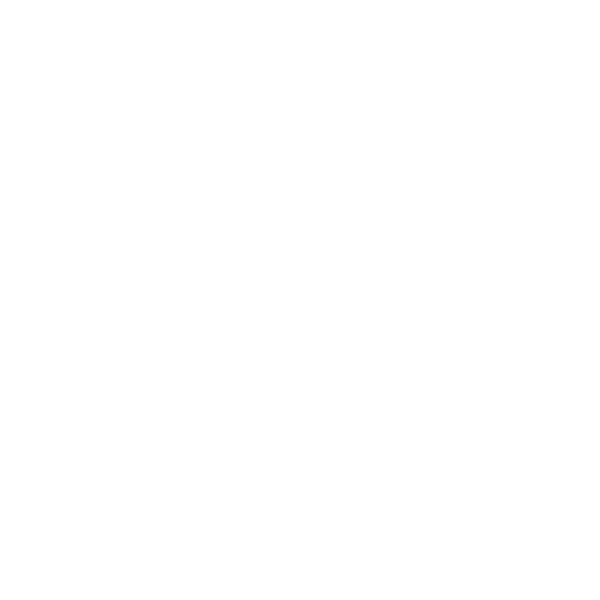# 2022世界杯预选赛12强燃料的一种含有100块的碳合金，包括ART的ARTATAT的SSSSSSSSSSSSSSSS？

## 1。轮胎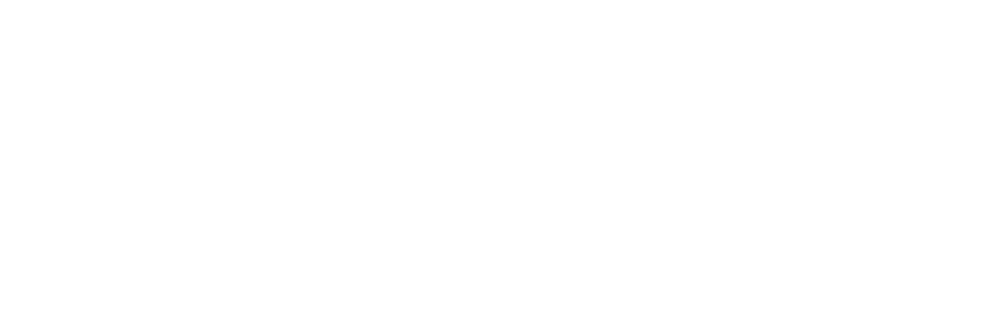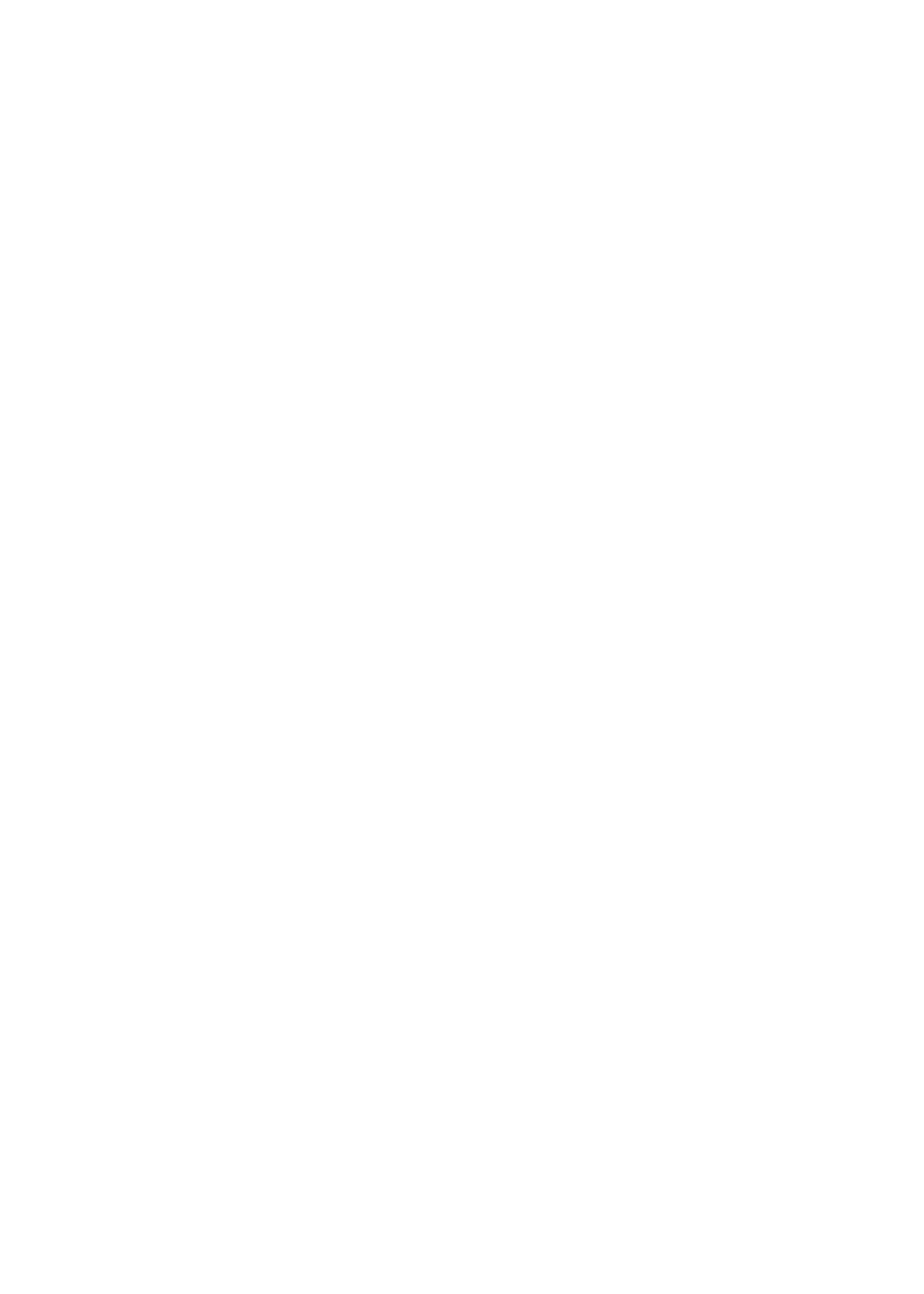### 细胞细胞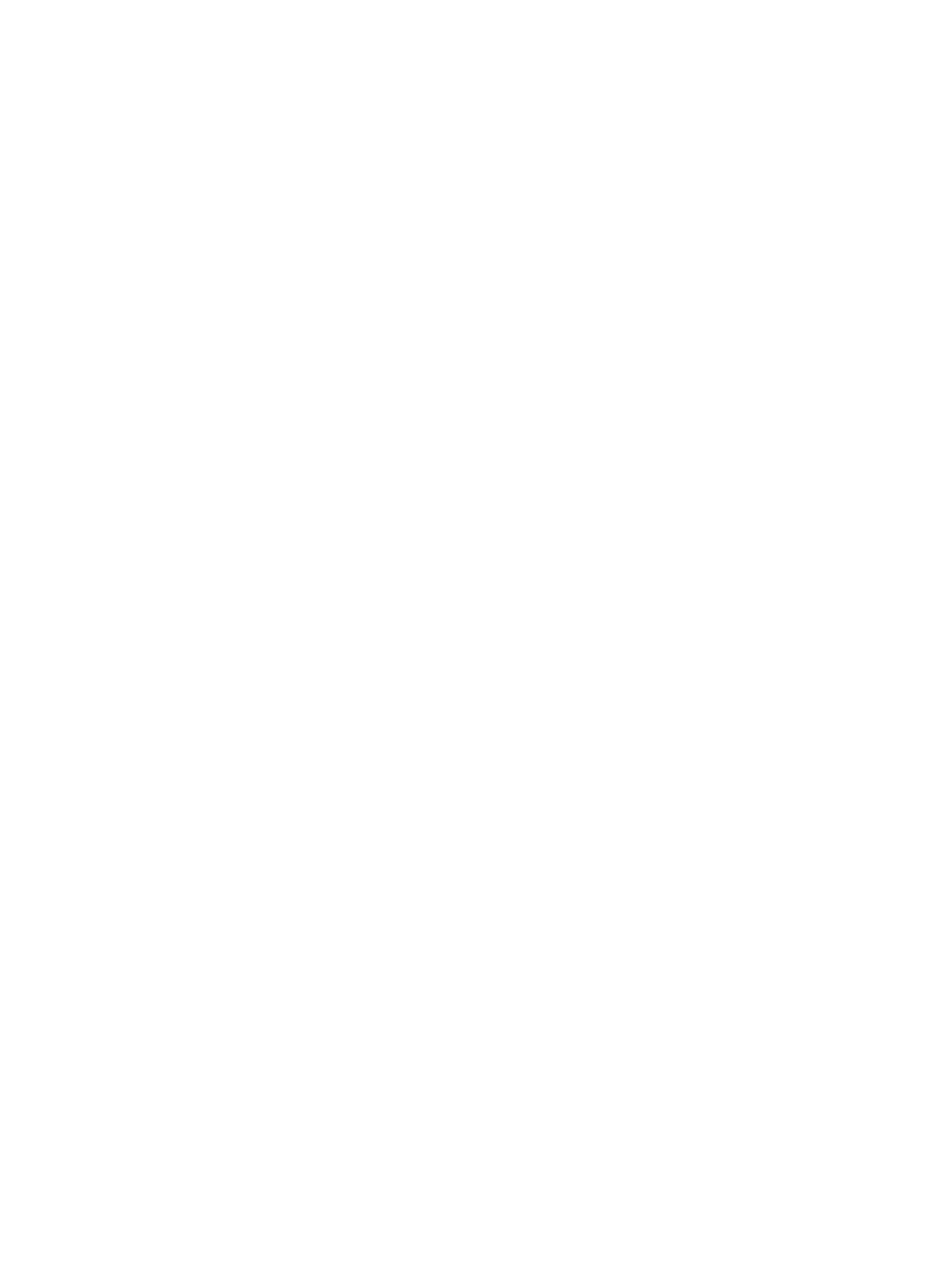### 激光纤维停止了……无法抑制的肌肉损伤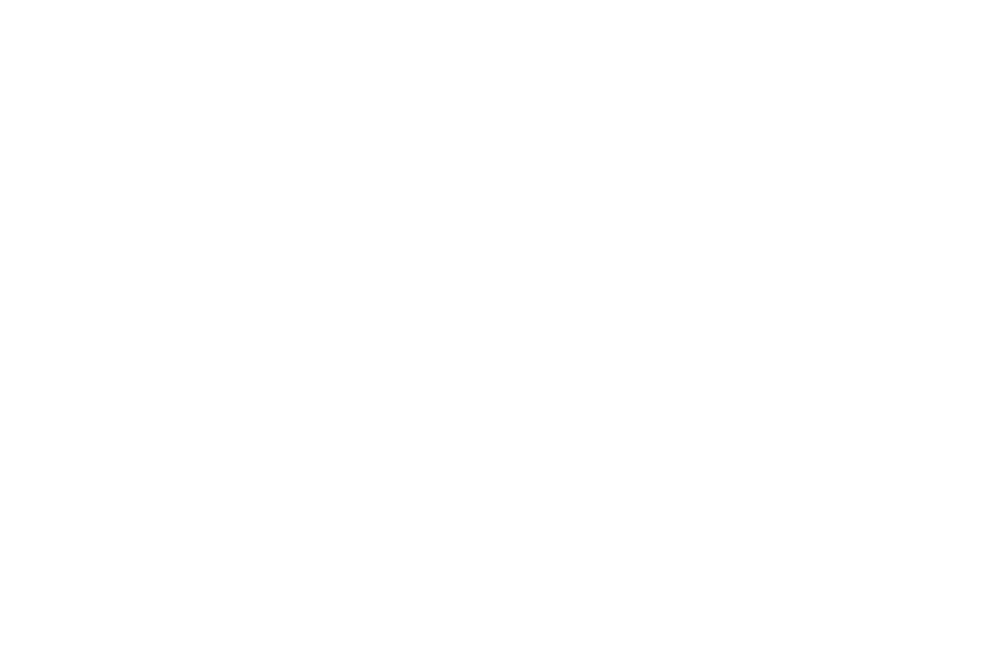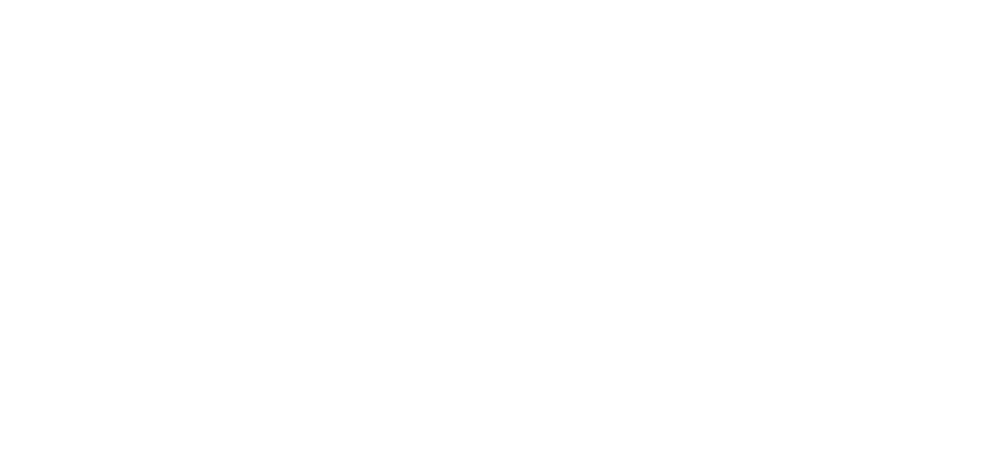## 照片：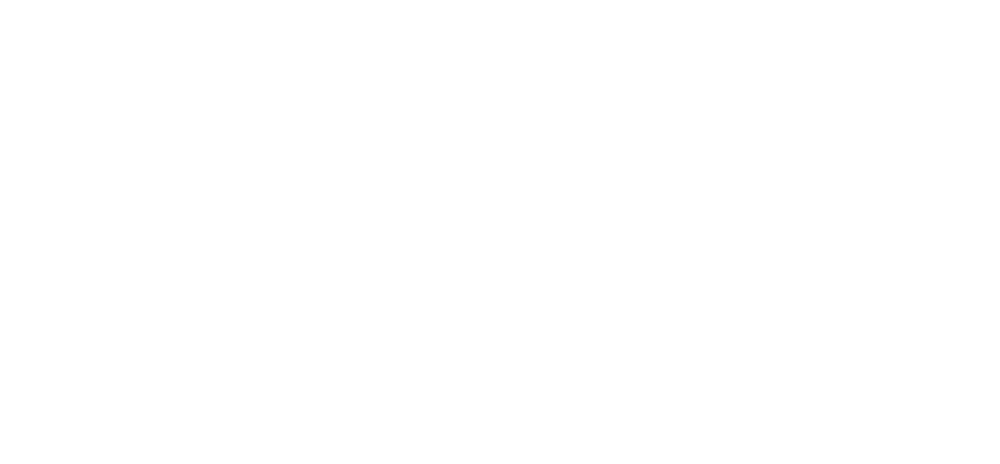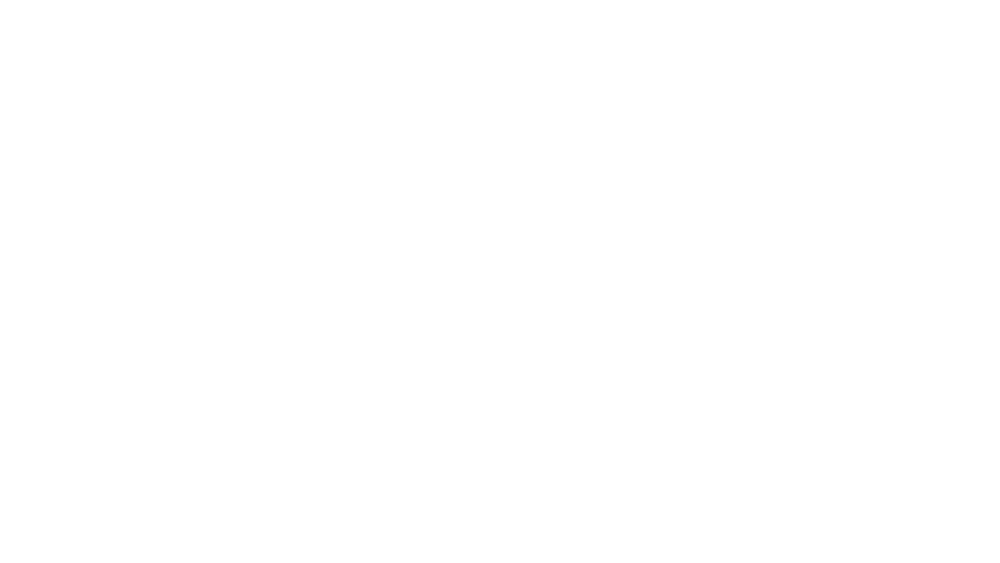## 照片：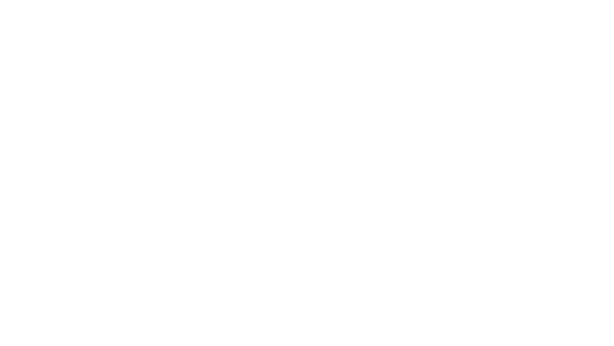## 照片：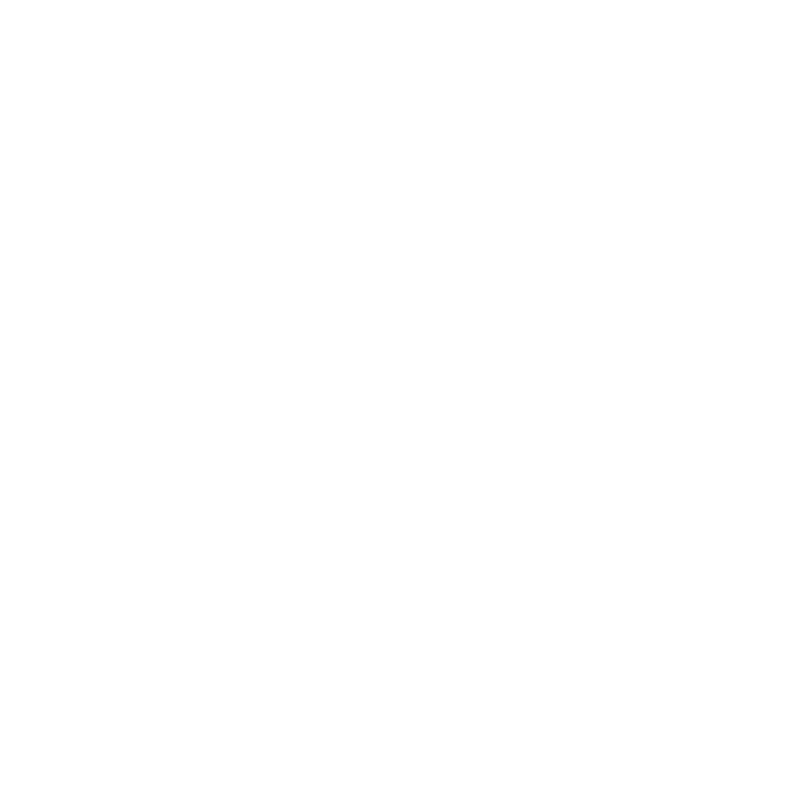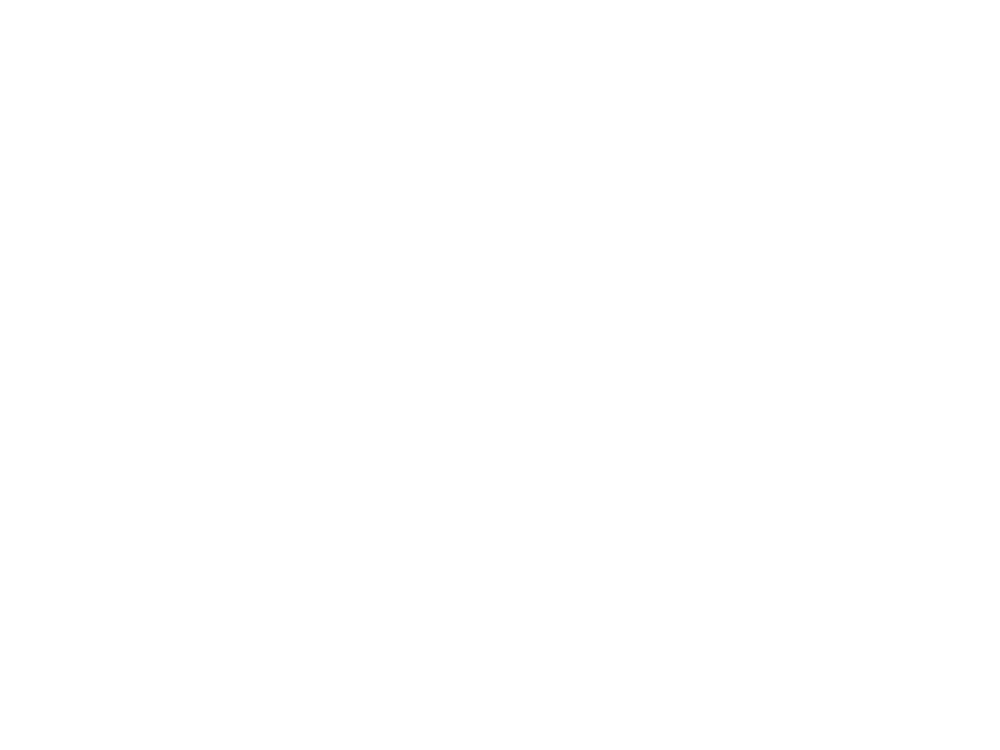## 照片：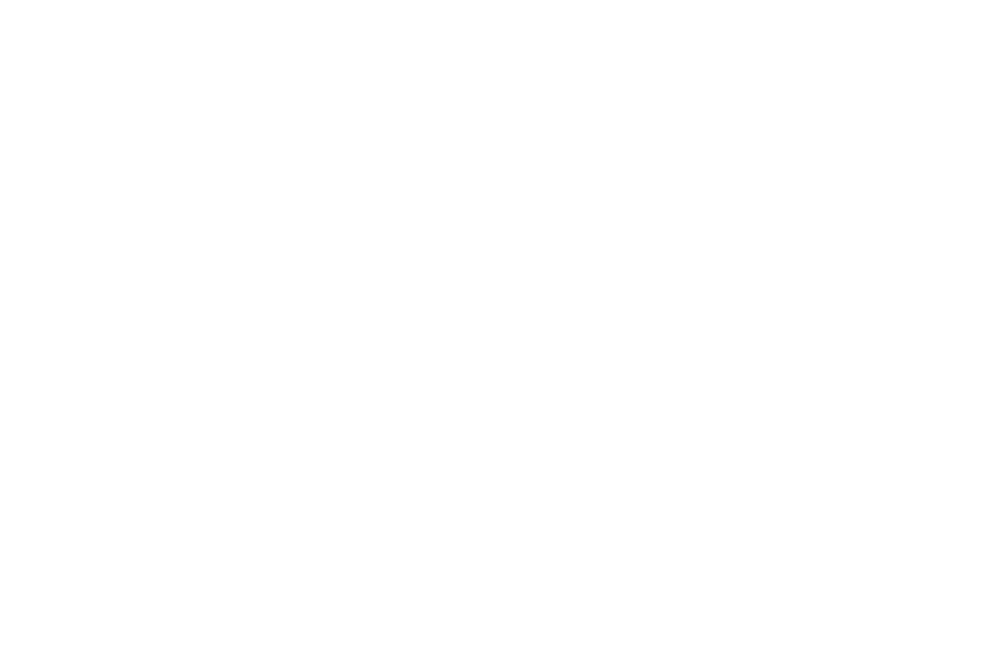## 照片：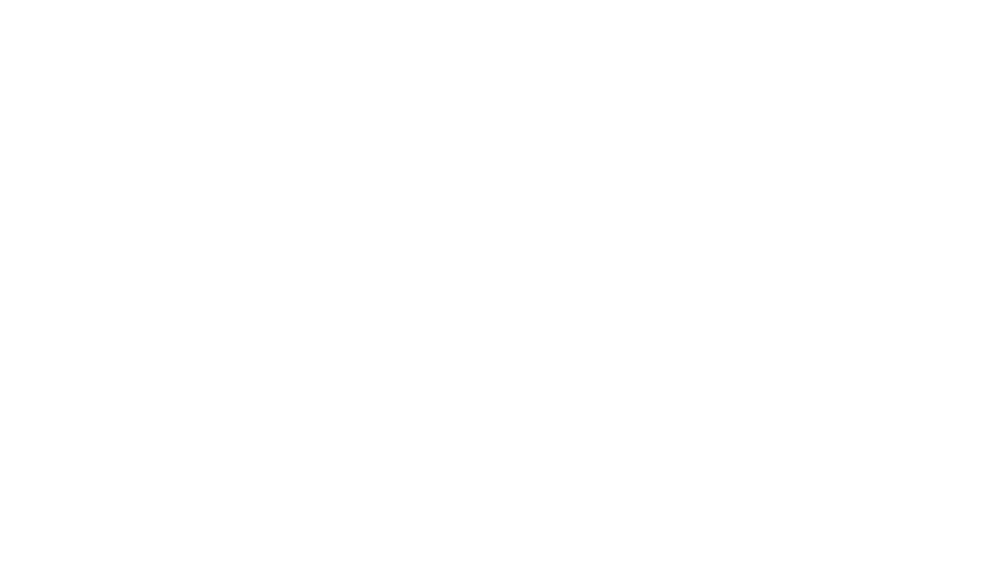## 照片：：：“288”，227号，216号公路，56760-21指纹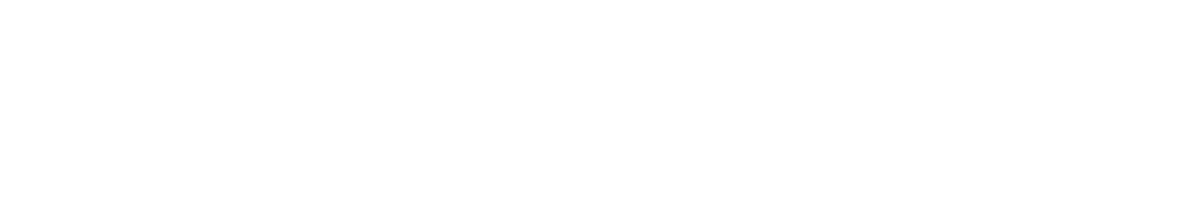### 你能做什么我们

#### 我们两小时后就会回复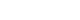来我们 我们两小时内就会有两个小时，但所有信息都会安全的。